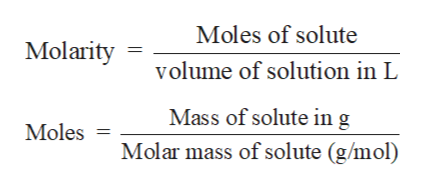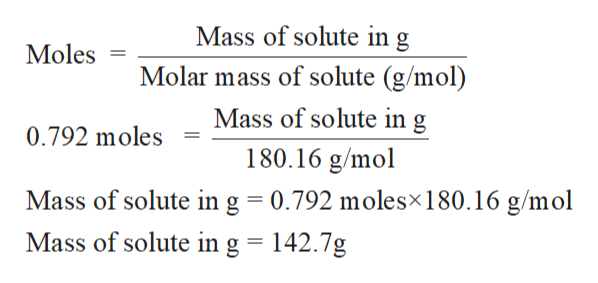# Whatis the molarity of 0.792mof C6H12O6, if the solution density is 1.16 g/mL. Molar mass (C6H12O6)= 180.16 g

Question
Whatis the molarity of 0.792mof C6H12O6, if the solution density is 1.16 g/mL. Molar mass (C6H12O6)= 180.16 g
check_circle

star
star
star
star
star
1 Rating
Step 1

Solution stoichiometry is mainly based on the calculation of moles and volumes. These two values are used to calculate the molarity of solution. The relation between moles, volume and molarity is as given below;help_outlineImage TranscriptioncloseMoles of solute Molarity volume of solution in L Mass of solute in g Moles Molar mass of solute (g/mol) fullscreen
Step 2

The molality can be defined as the number of moles of solute dissolve in kg of the solvent. The molality can be given below;

Step 3

Molality  = 0.792 m

Density  = 1.16 g/mL

0.792 m means 0.792 moles of glucose is...help_outlineImage TranscriptioncloseMass of solute in g Molar mass of solute (g/mol) Moles Mass of solute in 0.792 moles 180.16 g/mol Mass of solute ing 0.792 molesx180.16 g/mol Mass of solute in g = 142.7g fullscreen

### Want to see the full answer?

See Solution

#### Want to see this answer and more?

Solutions are written by subject experts who are available 24/7. Questions are typically answered within 1 hour.*

See Solution
*Response times may vary by subject and question.
Tagged in

### Solutions